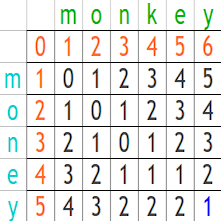# Edit Distance and Jaccard Distance Calculation with NLTK

Tutorial Contents

## Edit Distance

Edit Distance (a.k.a. Levenshtein Distance) is a measure of similarity between two strings referred to as the source string and the target string.The distance between the source string and the target string is the minimum number of edit operations (deletions, insertions, or substitutions) required to transform the source into the target. The lower the distance, the more similar the two strings.

Among the common applications of the Edit Distance algorithm are: spell checking, plagiarism detection, and translation memory systems.

### Edit Distance Python NLTK

NLTK library has the Edit Distance algorithm ready to use. Let’s take some examples.

#### Example #1

The output is 1 because the difference between “mapping” and “mappings” is only one character, “s”.

#### Example #2

Basic Spelling Checker: Let’s assume you have a mistaken word and a list of possible words and you want to know the nearest suggestion.

You will get the output:

As you can see, comparing the mistaken word “ligting” to each word in our list,  the least Edit Distance is 1 for words: “listing” and “lighting” which means they are the best spelling suggestions for “ligting”. Yes, a smaller Edit Distance between two strings means they are more similar than others.
Bonus: You can have list of words from several sources, such as:
• NLTK:  words = nltk.corpus.words.words()
• Check answers of this question.
• Check lists at Kaggle.
• Google: Search for “list of English words”.

#### Example #3

Sentence or paragraph comparison is useful in applications like plagiarism detection (to know if one article is a stolen version of another article), and translation memory systems (that save previously translated sentences and when there is a new untranslated sentence, the system retrieves a similar one that can be slightly edited by a human translator instead of translating the new sentence from scratch).

You will get the output:

So it is clear that sent1 and sent2 are more similar to each other than other sentence pairs.

## Jaccard Distance

Jaccard Distance is a measure of how dissimilar two sets are.  The lower the distance, the more similar the two strings.
Jaccard Distance depends on another concept called “Jaccard Similarity Index” which is (the number in both sets) / (the number in either set) * 100
Then we can calculate the Jaccard Distance as follows:

For example, if we have two strings: “mapping” and “mappings”, the intersection of the two sets is 6 because there are 7 similar characters, but the “p” is repeated while we need a set, i.e. unique characters, and the union of the two sets is 7, so the Jaccard Similarity Index is 6/7 = 0.857 and the Jaccard Distance is 1 – 0.857 = 0.142

### Jaccard Distance Python NLTK

The good news is that the NLTK library has the Jaccard Distance algorithm ready to use. Let’s take some examples.

#### Example #1

Unlike Edit Distance, you cannot just run Jaccard Distance on the strings directly; you must first convert them to the set type.

#### Example #2

Basic Spelling Checker: It is the same example we had with the Edit Distance algorithm; now we are testing it with the Jaccard Distance algorithm. Let’s assume you have a mistaken word and a list of possible words and you want to know the nearest suggestion.

You will get the output:

Again, comparing the mistaken word “ligting” to each word in our list,  the least Jaccard Distance is 0.166 for words: “listing” and “lighting” which means they are the best spelling suggestions for “ligting” because they have the lowest distance.

#### Example #3

If you are wondering if there is a difference between the output of Edit Distance and Jaccard Distance, see this example.

You will get the result:

Just like when we applied Edit Distance, sent1 and sent2 are the most similar sentences. However, look to the other results; they are completely different. The most obvious difference is that the Edit Distance between sent1 and sent4 is 32 and the Jaccard Distance is zero, which means the Jaccard Distance algorithms sees them as identical sentence because Edit Distance depends on counting edit operations from the start to end of the string while Jaccard Distance just counts the number characters and then apply some calculations on this number as mentioned above. Actually, there is no “right” or “wrong” answer; it all depends on what you really need to do.

## Tokenization

If you want to work on word level instead of character level, you might want to apply tokenization first before calculating Edit Distance and Jaccard Distance. This can be useful if you want to exclude specific sort of tokens or if you want to run some pre-operations like lemmatization or stemming.

## n-gram

In general, n-gram means splitting a string in sequences with the length n. So if we have this string “abcde”, then bigrams are: ab, bc, cd, and de while trigrams will be: abc, bcd, and cde while 4-grams will be abcd, and bcde.

n-grams can be used with Jaccard Distance. n-grams per se are useful in other applications such as machine translation when you want to find out which phrase in one language usually comes as the translation of another phrase in the target language.

Back to Jaccard Distance, let’s see how to use n-grams on the string directly, i.e. on the character level, or after tokenization, i.e. on the token level.

#### Example #2: Token Level

You can run the two codes and compare results. Again, choosing which algorithm to use all depends on what you want to do.

If you have questions, please feel free to write them in a comment below.